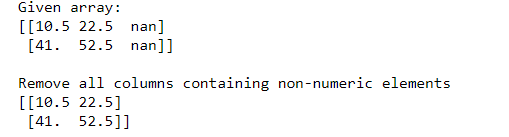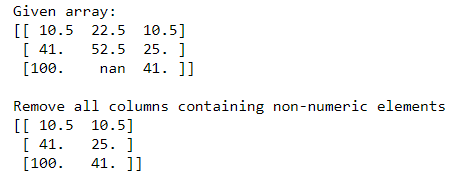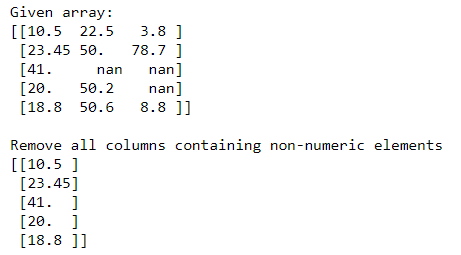Related Articles

# How to Remove columns in Numpy array that contains non-numeric values?

• Last Updated : 25 Oct, 2020

Many times we have non-numeric values in NumPy array. These values need to be removed, so that array will be free from all these unnecessary values and look more decent. It is possible to remove all columns containing Nan values using the Bitwise NOT operator and np.isnan() function.

Example 1:

## Python3

 `# Importing Numpy module``import` `numpy as np`` ` `# Creating 2X3 2-D Numpy array``n_arr ``=` `np.array([[``10.5``, ``22.5``, np.nan],``                  ``[``41``, ``52.5``, np.nan]])`` ` `print``(``"Given array:"``)``print``(n_arr)`` ` `print``(``"\nRemove all columns containing non-numeric elements "``)``print``(n_arr[:, ~np.isnan(n_arr).``any``(axis``=``0``)])`

Output:In the above example, we remove columns containing non-numeric values from the 2X3 Numpy array.

Example 2:

## Python3

 `# Importing Numpy module``import` `numpy as np`` ` `# Creating 3X3 2-D Numpy array``n_arr ``=` `np.array([[``10.5``, ``22.5``, ``10.5``],``                  ``[``41``, ``52.5``, ``25``],``                  ``[``100``, np.nan, ``41``]])`` ` `print``(``"Given array:"``)``print``(n_arr)`` ` `print``(``"\nRemove all columns containing non-numeric elements "``)``print``(n_arr[:, ~np.isnan(n_arr).``any``(axis``=``0``)])`

Output:In the above example, we remove columns containing non-numeric values from the 3X3 Numpy array.

Example 3:

## Python3

 `# Importing Numpy module``import` `numpy as np`` ` `# Creating 5X3 2-D Numpy array``n_arr ``=` `np.array([[``10.5``, ``22.5``, ``3.8``],``                  ``[``23.45``, ``50``, ``78.7``],``                  ``[``41``, np.nan, np.nan],``                  ``[``20``, ``50.20``, np.nan],``                  ``[``18.8``, ``50.60``, ``8.8``]])`` ` `print``(``"Given array:"``)``print``(n_arr)`` ` `print``(``"\nRemove all columns containing non-numeric elements "``)``print``(n_arr[:, ~np.isnan(n_arr).``any``(axis``=``0``)])`

Output:In the above example, we remove columns containing non-numeric values from the 5X3 Numpy array.

Attention geek! Strengthen your foundations with the Python Programming Foundation Course and learn the basics.

To begin with, your interview preparations Enhance your Data Structures concepts with the Python DS Course. And to begin with your Machine Learning Journey, join the Machine Learning – Basic Level Course

My Personal Notes arrow_drop_up